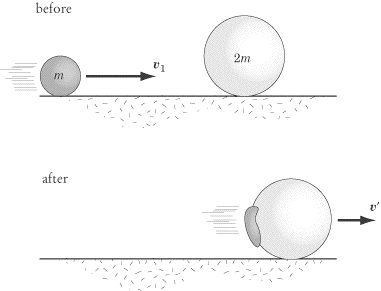# Momentum conservation problem

Lets a plastic ball of mass m which is collided with steel. After collision the ball is coming back with the half initial speed. If the steel doesn't move then how can I interpretate this ?

Let the initial speed of the ball is $u_1$ and mass $m_1$ and mass of steel $m_2$ speed of steel before and after collision $0$.

Therefore we can write according to the conservation of momentum, $$m_1 u_1 +m_2 u_2 = m_1v_1 +m_2 v_2$$ $$m_1 u_1 = m_1v_1$$ $$u_1 = v_1$$

I have surmised $u_2 = v_2 = 0$.

Therefore speed is same then how can the speed of the ball can be halved after the collision. Is this because of inelastic collision?

• This cannot happen in this world as you are violating the law of conservation of momentum here. Dec 6, 2013 at 10:07

If "the steel" that you're referring to is a fixed object or has a large mass, then the energy lost in the collision goes into increasing the internal thermal energy of the plastic ball and "the steel". This is an inelastic collision and kinetic energy is never conserved in inelastic collisions. Momentum, on the other hand is always conserved in the collision, but as your condition states, "the steel does not move". It means some other particle in the universe is gaining the momentum lost by the particle, or "the steel" moves so slowly that you are unable to measure it, or something else...

• If someone asks that, what force experience the ball during collision if after the collision speed of the ball reduced to half. Dec 6, 2013 at 6:33
• @user2378: force is just the rate of change of momentum. So, the force=(m1v1-m1u1)/time Dec 6, 2013 at 9:08
• Time is not mentioned here. Read the question again. Dec 6, 2013 at 9:09

If the steel doesn't move then how can I interpretate this ?

The interpretation you mentioned is completely wrong.(why?)

Therefore speed is same then how can the speed of the ball can be halved after the collision. Is this because of inelastic collision?

No!. Consider the steel is initially at rest and the plastic ball is moving towards it. If the collision is taken place only between these two bodies ( i.e system under consideration is isolated) then even if the collision is inelastic the velocity of the steel(was at rest before collision) which is been hit after the collision can not be $0$ even if it were a completely inelastic collision.The reason why after the collision velocity of steel should not be $0$ is that when the collision takes place both the bodies impart equal and opposite forces on each other.Since a force is acting upon the steel it will increase the velocity from $0$( because initially steel was at rest) to a certain value.

Then how should we interpret the collision.

Right interpretation

Let us suppose the system is isolated then the momentum of the system should remain conserved i.e
$$m_1 u_1 +m_2 u_2 = m_1v_1 +m_2 v_2$$ $$\Longrightarrow m_1u_1=m_1v_1$$ $$\Longrightarrow u_1=v_1$$

This result is contrary to the observation($u_1=-1/2 v_1$). This implies that our assumption that the system is isolated is wrong.
A better interpretation is that the system under consideration contains more than two bodies. For example the steel might be placed right behind another object(of same mass as that of steel) in such a way that when the collision occurs the plastic body impart some force on steel and steel acquires a velocity but suddenly this steel collides with the third body of same mass as that of steel elastically. Now the collision of steel with third body will cause the steel to stop momentarily and the third body will start moving with the speed with which the steel should have been moving. Also since the steel was placed right behind the third body it appears as the steel remains stationary all along.

• I have got you but how can I evaluate the force experienced by the ball without knowing the time? Let we have the ball of 2 kg and the initial velocity is $50ms^{-1}$ Dec 6, 2013 at 18:35
• @user2378 in any usual collision force acts between the two bodies for a relatively small interval of time. Moreover the force vary during the collision. all you can find is $∫_0^{ΔT}F.dt=Δp$. But for an idea say a average Force$F_av$ acts then $F_av.ΔT=Δp$ even now you need to know the time of collision to have an idea of average force. Dec 7, 2013 at 4:35

If $m_2 < \infty$ this is impossible. If $m_2 = \infty$ it is possible because $\infty \times 0$ is any number.

• Could you expand on the answer and explain why it fits the situation? Dec 6, 2013 at 13:39
• Consider a limiting case $m_2 \to \infty$. From conservation of momentum you see that velocity of steel after collision (SAC) $\propto 1/m_2$. Thus if $m_2 < \infty$ the initial statement cannot be valid (velocity of SAC cannot be $0$). However if $m_2$ becomes infinite, this velocity goes to zero, furthermore it does not depend on change of velocity of the ball. But you should notice that kinetic energy of SAC is $\propto m_2 (1/m_2)^2 \to 0$ so energy still is not conserved. Thus we have an inelastic collision with infinitely massive steel. Physically $m_2<\infty$ means that $m_1/m_2 \ll 1$.
– xaxa
Dec 6, 2013 at 15:12
• I meant that you should edit that into the answer :) Comment are temporary. Dec 6, 2013 at 15:22